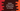# Python program to count the words and characters in a string## Introduction :

In this python programming tutorial, we will learn how to count the total words and characters in a string. The program will take the string as input from the user, count the words and characters and then it will print out the result.

## Algorithm :

Following algorithm we will use in this program :

1. Ask the user to enter a string.
2. Split the string into words and save it in a variable.
3. Calculate the total number of split words, i.e. the total count of words in the string.
4. Iterate over the words one by one. For each word, count the character and keep adding to a final variable.
5. Print out the total count of words and characters to the user.

## Program to count the words and characters in a string:

``````#1
word_count = 0
char_count = 0

#2
usr_input = input("Enter a string : ")

#3
split_string = usr_input.split()

#4
word_count = len(split_string)

#5
for word in split_string:
#6
char_count += len(word)

#7
print("Total words : {}".format(word_count))
print("Total characters : {}".format(char_count))``````

### Explanation :

The commented numbers in the above program denote the step numbers below :

1. Create two variables to store the final word count and character count: wordcount_ and charcount. Initialize them with value _0.
2. Ask the user to enter a string. Read and store the string in a usrinput_ variable.
3. Split the user-provided string using the split() method. It will break the string at all white space and return one list holding these sub-strings.
4. Count the number of words in the split string. Store it in the variable wordcount_.
5. Now, using one for loop, iterate over the words in the split words list.
6. While iterating, add the length of each word to the variable charcount_.
7. The word count is stored in the wordcount_ variable and character count is in charcount_. Print out the count results to the user.

### Sample Output :

``````Enter a string : Hello world
Total words : 2
Total characters : 10

Enter a string : The quick brown fox jumps over the lazy dog
Total words : 9
Total characters : 35

Enter a string : abc def gh ij k l mno pqr stu vwx yz
Total words : 11
Total characters : 26

Enter a string : a b c d e f g h i j k l m n o p q r s t u v w x y z
Total words : 26
Total characters : 26``````

This program is also available on [Github](https://github.com/codevscolor/codevscolor/blob/master/python/countwordscharinstr.py). Send us a pull request if you have a better solution.

### Conclusion :

In this tutorial, we have learned how to split a string into words and how to calculate the total words and characters in a string. Counting words in a string is one of the most commonly used programs in Python development. You can use the same program to count the total number of words in a file. Try to go through the code shown above and drop one comment below if you have any queries.